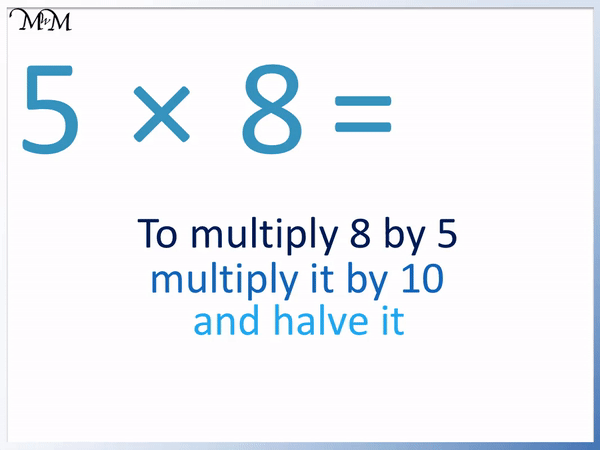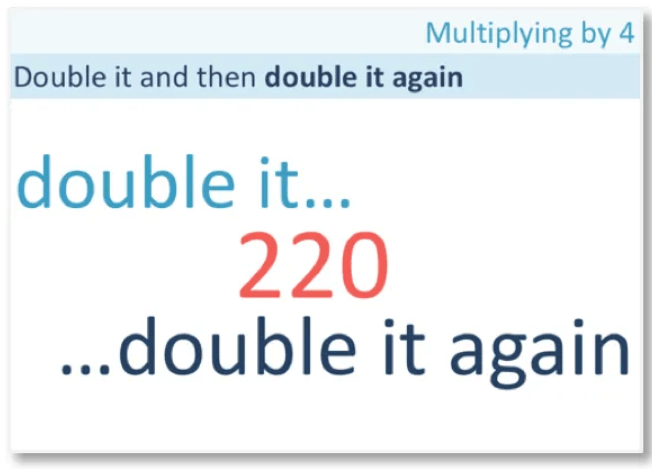# The 8 Times Table

The 8 Times TableThe 8 times table is:

• 1 × 8 = 8
• 2 × 8 = 16.
• 3 × 8 = 24
• 4 × 8 = 32
• 5 × 8 = 40
• 6 × 8 = 48
• 7 × 8 = 56
• 8 × 8 = 64
• 9 × 8 = 72
• 10 × 8 = 80
• 11 × 8 = 88
• 12 × 8 = 96• The 8 times table is made by counting up in eight.
• The numbers in the eight times table repeat the pattern of ending in 8, 6, 4, 2 and 0.
• To multiply a number by 8, we can double it, double it and double it again.

The numbers in the eight times table repeat the pattern of ending in 8, 6, 4, 2 and 0.• Here is 12 × 8.
• To multiply 8 by 12, we multiply 8 by 10 and 2 separately and add the results together.
• 10 × 8 = 80.
• 2 × 8 = 16.
• 80 + 16 = 96 and so, 12 × 8 = 96.#### 8 Times Table Flashcards

Click on the 8 times table flashcards below to memorise the 8 times table.

×
×

=

# The 8 Times Table

## What are the 8 Times Tables?

The 8 times table is:

• 1 × 8 = 8
• 2 × 8 = 16.
• 3 × 8 = 24
• 4 × 8 = 32
• 5 × 8 = 40
• 6 × 8 = 48
• 7 × 8 = 56
• 8 × 8 = 64
• 9 × 8 = 72
• 10 × 8 = 80
• 11 × 8 = 88
• 12 × 8 = 96

Here is the complete 8 times table chart.## How to Learn the 8 Times Table

To learn the 8 times table, remember that the numbers end in the repeating pattern of 8, 6, 4, 2 and 0.

We can group the 8 times table chart into rows of five to see this times table pattern more easily.If we know a number in the 8 times table, we can figure out the next number along using this pattern. For example, if we know that 2 × 8 = 16, then we can figure out 3 × 8. 16 ends in 6 and the next number in the pattern 8, 6, 4, 2, 0 after 6 is 4. Therefore 3 × 8 ends in a 4. 3 × 8 = 24.

Apart from going from 5 × 8 = 40 to 6 × 8 = 48 and going from 10 × 8 = 80 to 11 × 8 = 88, all other numbers in the 8 times table increase their tens digit from one number to the next.

So if we remember that 8 × 8 = 64, then we can figure out 9 × 8. Firstly, 64 ends in a 4 and the next number in the pattern of 8, 6, 4, 2, 0 after 4 is a 2. This means that 9 × 8 ends in a 2. We also know that 64 starts with a 6 and so 9 × 8 starts with the number after 6, which is 7. Therefore 9 × 8 = 72.

We simply add eight to get from one number in the 8 times table to the next. One easy way to do this is to add 10 and then subtract 2.

Another pattern can be seen in the 8 times table when it is arranged into rows of five.The eight times table trick is that when multiplying 8 by a number from 1 to 5, the answer starts with a digit that is one less than the number 1 to 5. When multiplying 8 by a number from 6 to 10, the answer starts with a digit that is two less than the number from 6 to 10. When multiplying 8 by 11 or 12, the answer starts with a digit that is three less than either 11 or 12.

By arranging the 8 times table chart into rows of five, we can see this pattern in the columns.

The numbers in the first column start with a digit that is 1 less than the number we are multiplying by.

The numbers in the second column start with a digit that is 2 less than the number we are multiplying by.

The numbers in the third column start with a digit that is 3 less than the number we are multiplying by.

Whilst it is best to learn the eight times table by heart, this trick can be a useful starting point for remembering the times table when first learning it. It can be a useful prompt for a child first learning the 8 times table.

## Examples of the 8 Times Table

The eight times table is one of the more challenging times tables to learn because the numbers are relatively large compared with other times tables. There are some simple tricks to learning the 9, 10 and 11 times table and so, the 8 times table is one of the larger times tables without an easy pattern.

To remember the 8 times table it helps to link it with tricks found in the other times tables. Therefore we recommend that you learn the 8 times table after some of the other times tables have been mastered, or in conjunction with other times tables. This is because learning one times table reinforces another.

The 2 times table should be one of the first times tables learnt because it involves doubling.

To double, we simply add a number to itself.8 + 8 = 16 and so, 2 × 8 = 16.

Next we can learn 10 × 8 = 80 using the ten times table.To multiply a whole number by 10, we simply put a zero on the end and so, 10 × 8 = 80.

We can find 11 × 8 = 88 because to multiply a single digit number by 11 simply repeat the digit.

We repeat the digit 8.If we know that 10 × 8 = 80, we can easily find 5 × 8 by halving this answer.

Half of 80 is 40.

Therefore if 10 × 8 = 80,then 5 × 8 = 40.To multiply a single digit number by 9, we can subtract one from this number to see what the answer starts with. We then know that the digits of the answer must add to make 9.

So to multiply 8 by 9, subtract 1 from 8 to see that it starts with a 7.

7 + 2 = 9 and so, 9 × 8 = 72.There is a trick for multiplying the even digits of 2, 4, 6 and 8 by 6. This means that we can remember 6 × 8.

We write down the even digit being multiplied by 6 as the units digit of the answer. We then halve this digit to find the tens digit that the answer starts with.

8 is the units digit and half of 8 is 4, which is the tens digit.6 × 8 = 48.

Finally, multiplying numbers by 12 can be more challenging due to the larger numbers involved.

It can help to multiply the number by 10 and then by 2 before adding these results together.

10 × 8 = 80 and 2 × 8 = 16.

Multiplying by 10 and 2 is relatively easy using the tricks seen above.

We then add 80 + 16 to get 96.12 × 8 = 96.

These tricks can help us remember the 8 times table a bit more fluently.

Because 8 is made up of 2 × 2 × 2, we can use another trick of doubling, doubling and doubling again to multiply a number by 8.

For example to work out 3 × 8, we double 3, double the result and double this result again.

3 × 2 = 6 and 6 × 2 = 12 and 12 × 2 = 24.

Therefore 3 × 8 = 24.Now try our lesson on Multiplying by 4 and 8 by Doubling where we learn how to use doubling to multiply by 4 or 8.error: Content is protected !!